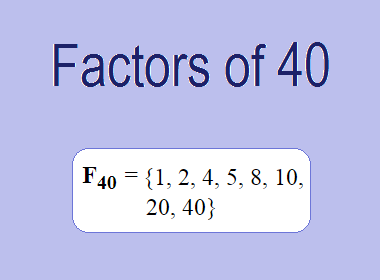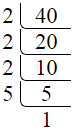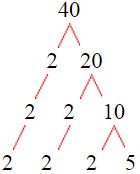# Factors of 40The factors of 40 are 1, 2, 4, 5, 8, 10, 20, and 40 i.e. F40 = {1, 2, 4, 5, 8, 10, 20, 40}. The factors of 40 are all the numbers that can divide 40 without leaving a remainder.

We can check if these numbers are factors of 40 by dividing 40 by each of them. If the result is a whole number, then the number is a factor of 40. Let's do this for each of the numbers listed above:

·        1 is a factor of 40 because 40 divided by 1 is 40.

·        2 is a factor of 40 because 40 divided by 2 is 20.

·        4 is a factor of 40 because 40 divided by 4 is 10.

·        5 is a factor of 40 because 40 divided by 5 is 8.

·        8 is a factor of 40 because 40 divided by 8 is 5.

·        10 is a factor of 40 because 40 divided by 10 is 4.

·        20 is a factor of 40 because 40 divided by 20 is 2.

·        40 is a factor of 40 because 40 divided by 40 is 1.

## How to Find Factors of 40?

1 and the number itself are the factors of every number. So, 1 and 40 are two factors of 40. To find the other factors of 40, we can start by dividing 40 by the numbers between 1 and 40. If we divide 40 by 2, we get a remainder of 0. Therefore, 2 is a factor of 40. If we divide 40 by 3, we get a remainder of 1. Therefore, 3 is not a factor of 40.

Next, we can check if 4 is a factor of 40. If we divide 40 by 4, we get a remainder of 0. Therefore, 4 is also a factor of 40. We can continue this process for all the possible factors of 40.

Through this process, we can find that the factors of 40 are 1, 2, 4, 5, 8, 10, 20, and 40. These are the only numbers that can divide 40 without leaving a remainder.

********************

********************

## Properties of the Factors of 40

The factors of 40 have some interesting properties. One of the properties is that the sum of the factors of 40 is equal to 90. We can see this by adding all the factors of 40 together:

1 + 2 + 4 + 5 + 8 + 10 + 20 + 40 = 90

Another property of the factors of 40 is that the prime factors of 40 are 2 and 5 only.

## Applications of the Factors of 40

The factors of 40 have several applications in mathematics. One of the applications is in finding the highest common factor (HCF) of two or more numbers. The HCF is the largest factor that two or more numbers have in common. For example, to find the HCF of 40 and 48, we need to find the factors of both numbers and identify the largest factor they have in common. The factors of 40 are 1, 2, 4, 5, 8, 10, 20, and 40. The factors of 48 are 1, 2, 3, 4, 6, 8, 12, 16, 24, and 48. The largest factor that they have in common is 8. Therefore, the HCF of 40 and 48 is 8.

Another application of the factors of 40 is in prime factorization. Prime factorization is the process of expressing a number as the product of its prime factors. The prime factors of 40 are 2 and 5 only. Therefore, we can express 40 as:

40 = 2 × 2 × 2 × 5

We can do prime factorization by division and factor tree method also. Here is the prime factorization of 40 by division method,40 = 2 × 2 × 2 × 5

Here is the prime factorization of 40 by the factor tree method,40 = 2 × 2 × 2 × 5

## Conclusion

The factors of 40 are the numbers that can divide 40 without leaving a remainder. The factors of 40 are 1, 2, 4, 5, 8, 10, 20, and 40. The factors of 40 have some interesting properties, such as having a sum of 90. The factors of 40 have several applications in mathematics, such as finding the highest common factor and prime factorization.